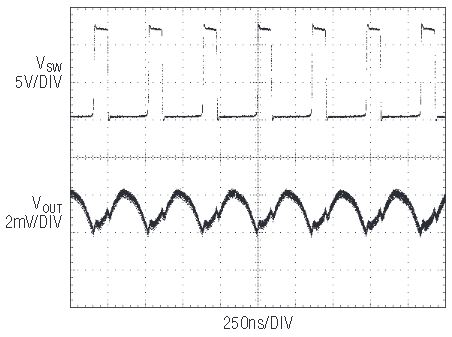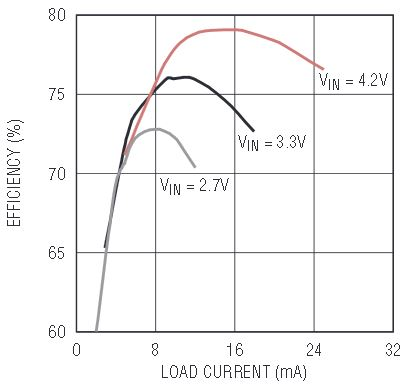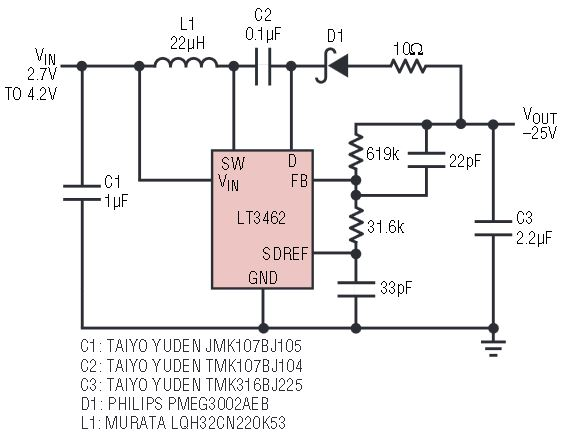# Tiny, Low Noise Boost and Inverter Solutions

Introduction

The LT3461 and LT3461A are current mode boost converters which combine a 40V rated, 1Ω NPN power switch with a power Schottky diode in a 6-lead ThinSOT package. This level of integration is unmatched by any currently available boost converter. The LT3462 and LT3462A are current mode inverters that offer the same level of integration.

Converters with outputs up to ±38V can be built on a very small footprint, making these parts ideal for compact display or imaging applications.

Everything about these devices focuses on squeezing high performance into the smallest spaces. The ‘A’ parts operate at high frequency—LT3461A boost switches at 3MHz; the LT3462A inverter at 2.7MHz—which allows the use of tiny, low profile components. For noise sensitive communication applications, the high, constant switching frequency results in low output voltage ripple and easily filtered switching harmonics. The non-’A’ parts run at 1.3MHz (LT3461) and 1.2MHz (LT3462) and are intended for applications which require high efficiency or high conversion ratios.

Furthermore, the internally-compensated current-mode PWM architecture minimizes the size and number of external parts, maximizes available output current and optimizes transient response.

Simple, Accurate Negative Regulators

It is easy to set the negative output voltage with the LT3462 and LT3462A inverting converters, because there is no need to compensate for a variable FB input bias current. The LT3462 and LT3462A feature a high impedance ground referenced FB input and a 2% accurate 1.262V reference output. An external resistor (R1) between the reference and the FB pin sets the current in the feedback divider. A second external resistor (R2) from the FB pin to the negative output sets the output voltage within 2% plus resistor tolerances. By eliminating the untrimmed current sourced by the negative FB (NFB) pin of other inverting regulators, calculation of the feedback divider has been simplified as follows.

The resulting output voltage is more accurate with less current flowing in the feedback divider.

–8V at 30mA in 50mm2

The 2.7MHz switching frequency of the LT3462A allows the use of tiny low profile inductors and low profile ceramic capacitors. Figure 1 shows a bias supply useful for CCD and OLED applications that produces a well regulated –8V supply at up to 30mA from 3.3V using as little as 50mm2 of board area. All components in this design are less than 1mm in height.Figure 1. Low profile, 3.3V to –8V, 30mA inverting converter in 50mm2.

Board area and profile are usually dominated by the inductor, which is usually the tallest component in the regulator and can occupy more area than the IC. Converters designed with the LT3462A do not have this limitation because the LT3462A works well with tiny, low profile inductors such as the Murata LQH2 series—with little impact on output power capability, and minimal impact on efficiency.

The –8V converter circuit also uses small (0805) low profile ceramic capacitors for the input, output and flying capacitors. An oscilloscope trace of the half load step on the output (Figure 2) shows these capacitors are sufficient to provide a well-damped transient response. The output voltage remains within 0.25% of the nominal value during the transient steps. Figure 3 shows the output voltage ripple of the –8V converter at 30mA is 2.2mVP–P.Figure 2. Transient response of the 3.3V-to-(– 8V) converter showing less than 0.25% total deviation with a 50% load step.Figure 3. Output ripple of the 3.3V-to-(–8V) inverter at 30mA is only 2.2mVP–P.

15V at 30mA in 50mm2

The 3MHz switching frequency of the LT3461A also allows the use of tiny, low profile components. Figure 4 shows a circuit that produces a well regulated 15V supply for CCD bias applications at up to 30mA from 3.3V using as little as 50mm2 of board area. All components in this design are also less than 1mm in height.Figure 4. Low profile, 3.3V to 15V, 30mA step-up converter occupies as little as 50mm2.

This circuit uses a low profile 2.2μF ceramic output capacitor for well-damped half load step transient response (Figure 5). The output voltage remains well within 1% of the nominal value during these transient steps. The choice of capacitor also impacts output voltage ripple. The output ripple of the circuit in Figure 4 at full load of 30mA is 10mVP-P, or less than 0.07% of the nominal 15V output.Figure 5. The transient response of the 3.3V-to-15V converter showing less than 120mV total deviation with a 50% load step.

Figure 6 shows that efficiency is better than 70% over a wide range of supply voltages and load currents.Figure 6. Efficiency of the 3.3V to 15V converter.

Optimizing for Efficiency

While the LT3461A (boost) and LT3462A (inverting) are optimized for small size, the LT3461 (boost) and LT3462 (inverting) are intended for applications requiring higher efficiencies or high conversion ratios. The lower switching frequencies translate to higher efficiencies because of a reduction in switching losses.

The LT3461 (boost) is guaranteed to a maximum switch duty cycle of 92% in continuous conduction mode, and the LT3462 (inverting) is guaranteed to a maximum switch duty cycle of 90%, which enables high conversion ratios at relatively high output currents.

Although high conversion ratios can also be obtained using discontinuous conduction mode (DCM)—where current in the inductor is allowed to go to zero each cycle—the DCM technique requires higher switch currents and larger inductors/rectifiers than a system operating in continuous conduction mode at the same load current. Because the LT3461 can switch at 1.3MHz in continuous conduction mode with up to 92% switch duty cycle, and the LT3462 at 1.2Mhz, 90% duty, they are the most compact solutions available for outputs 5 to 10 times the supply voltage. For example, the LCD bias circuit of Figure 7 provides 18mA at 25V from a 3.3V supply and occupies as little as 50mm2 of board space. Figure 8 shows that the efficiency of the 25V converter is quite good, peaking at 79% for a 4.2V supply. Figure 9 shows a 3.3V to –25V, 14mA inverter with efficiency above 70% (Figure 10).Figure 7. High conversion ratio, 3.3V to 25V step up converter occupies as little as 50mm2.Figure 8. Efficiency of the circuit in Figure 7 at 25V output.Figure 9. High conversion ratio, 3.3V to 25V inverting converter occupies as little as 55mm2.Figure 10. Efficiency of the circuit in Figure 9 at –25V output.

Conclusion

The LT3461, LT3461A, LT3462 and LT3462A provide very compact boost and inverter solutions for a wide input voltage range of 2.5V to 16V, and outputs to ±38V, making these devices a good fit in a variety of applications.